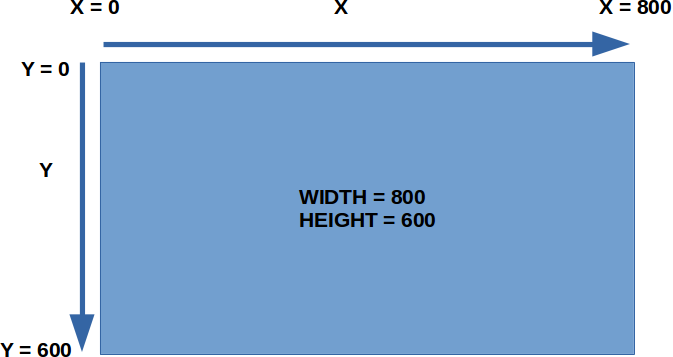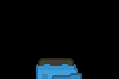# Player Tank

``````import pgzrun

WIDTH=800
HEIGHT=600

pgzrun.go() # Must be last line
``````

For now, keep your width and height at 800 and 600. Don't change it yet. This will be important later when we add in the walls.

Run (Press F5 or click "Run -> Run Module") and make sure it works.

Next, we're going to add a tank.

First, we'll create the tank actor...

``````tank = Actor('tank_blue')
``````

...then we create the draw() function, and add in a draw command for the tank.

``````def draw():
tank.draw()
``````

With that done, your program should now look like this...

``````import pgzrun

WIDTH=800
HEIGHT=600

tank = Actor('tank_blue')

def draw():
tank.draw()

pgzrun.go() # Must be last line
``````

Run it, and you should see your tank on screen.

# Position the tank

Now, let's have our tank start at the bottom of the screen, and turn it to face upwards.

Remember that to move the actor downwards, we need to increase y. The width of the screen is 800, so to put it in the center, we should set x to 400.Add in the following to your program. It should appear right after the line where you created the tank actor.

``````tank.y = 600
tank.x = 400
``````

Run it. Does the tank look right?It's cut off! When we set the position of the tank actor, it'll set it based on the center of the actor. Let's move it slightly upwards. Change the lines to...

``````tank.y = 575
tank.x = 400
``````

Try it. Better right?

### Rotation

To rotate the tank, we need to set an angle...

``````tank.angle = 90
``````

This will make the tank rotate 90 degrees clockwise. Now your program should look like this...

``````import pgzrun

WIDTH=800
HEIGHT=600

tank = Actor('tank_blue')
tank.y = 575
tank.x = 400
tank.angle = 90

def draw():
tank.draw()

pgzrun.go() # Must be last line
``````